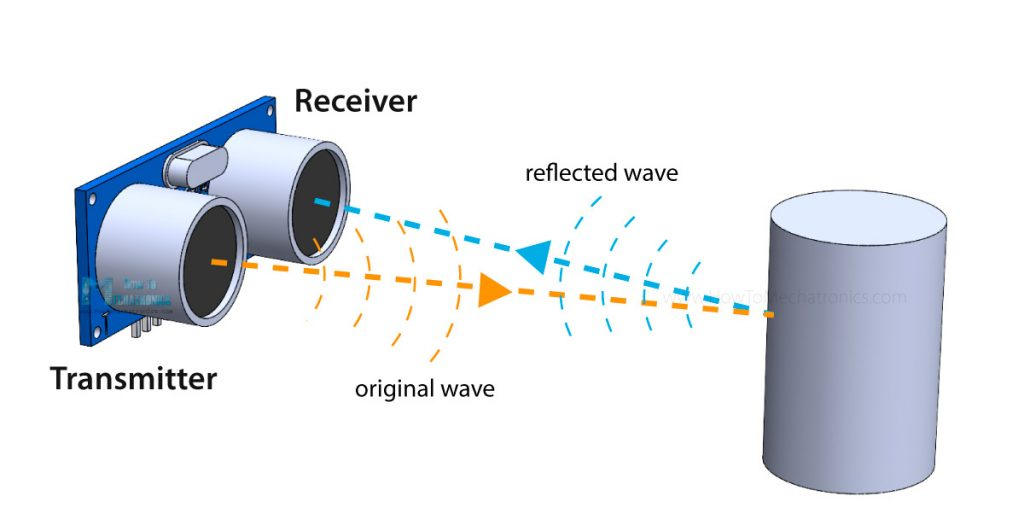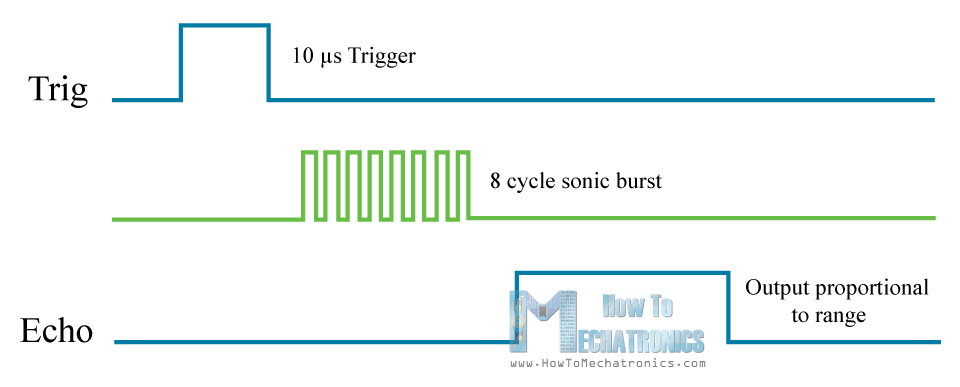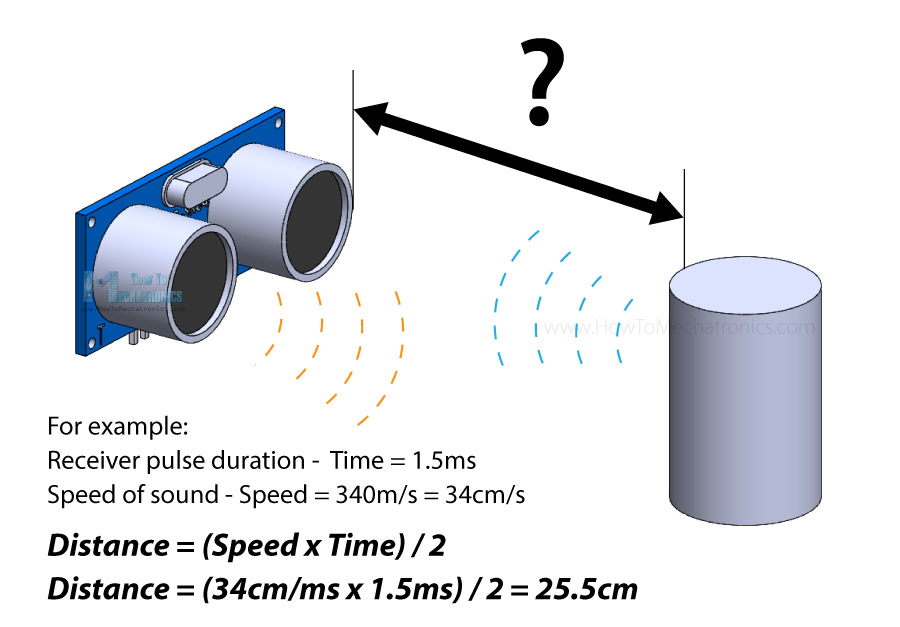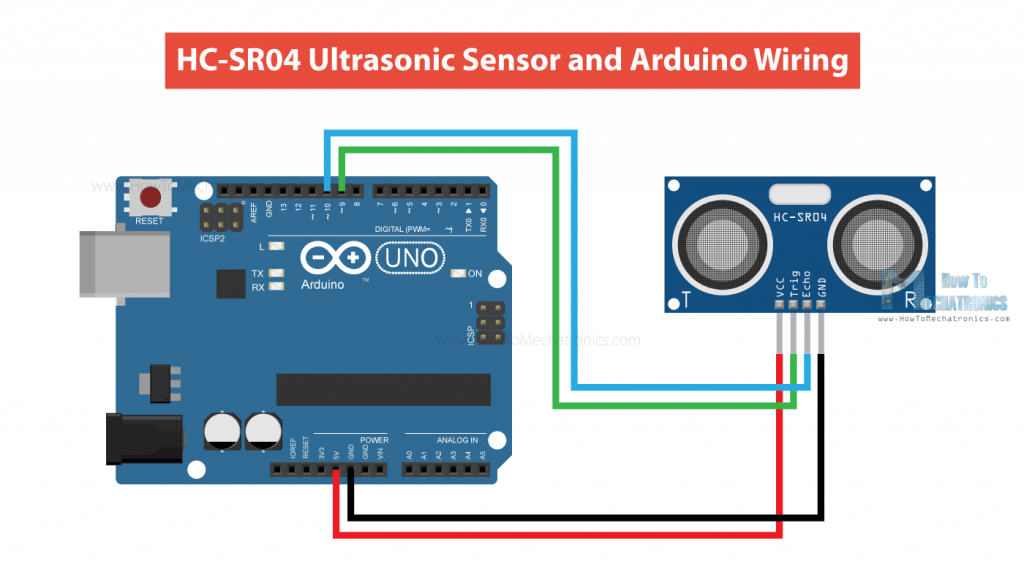Welcome to Ercoms Robotics Store | Lucknow!
0 0.00

No products in the cart.

# Ultrasonic Sensor (HC-SR04) Testion Using Arduino – Complete Guide

In this tutorial we will learn how the HC-SR04 ultrasonic sensor works and how to use it with Arduino. This is the most popular sensor for measuring distance and making obstacle avoiding robots with Arduino.

# HC-SR04 Hardware Overview

The HC-SR04 is an affordable and easy to use distance measuring sensor which has a range from 2cm to 400cm (about an inch to 13 feet).

The sensor is composed of two ultrasonic transducers. One is transmitter which outputs ultrasonic sound pulses and the other is receiver which listens for reflected waves. It’s basically a SONAR which is used in submarines for detecting underwater objects.

## Here are its main specifications:

 Operating Voltage 5V DC Operating Current 15mA Operating Frequency 40KHz Min Range 2cm / 1 inch Max Range 400cm / 13 feet Accuracy 3mm Measuring Angle <15° Dimension 45 x 20 x 15mm

## HC-SR04 Ultrasonic Sensor Pinout

Here’s the pinout of the sensor:

The sensor has 4 pins. VCC and GND go to 5V and GND pins on the Arduino, and the Trig and Echo go to any digital Arduino pin. Using the Trig pin we send the ultrasound wave from the transmitter, and with the Echo pin we listen for the reflected signal.

## How the HC-SR04 Ultrasonic Distance Sensor Works?

It emits an ultrasound at 40 000 Hz which travels through the air and if there is an object or obstacle on its path It will bounce back to the module. Considering the travel time and the speed of the sound you can calculate the distance.In order to generate the ultrasound we need to set the Trig pin on a High State for 10 µs. That will send out an 8 cycle ultrasonic burst which will travel at the speed of sound. The Echo pins goes high right away after that 8 cycle ultrasonic burst is sent, and it starts listening or waiting for that wave to be reflected from an object.

If there is no object or reflected pulse, the Echo pin will time-out after 38ms and get back to low state.If we receive a reflected pulse, the Echo pin will go down sooner than those 38ms. According to the amount of time the Echo pin was HIGH, we can determine the distance the sound wave traveled, thus the distance from the sensor to the object.

For that purpose we are using the following basic formula for calculating distance:

Distance = Speed x Time

We actually know both the speed and the time values. The time is the amount of time the Echo pin was HIGH, and the speed is the speed of sound which is 340m/s. There’s one additional step we need to do, and that’s divide the end result by 2. and that’s because we are measuring the duration the sound wave needs to travel to the object and bounce back.Let’s say the Echo pin was HIGH for 2ms. If we want the get the distance result in cm, we can convert the speed of sound value from 340m/s to 34cm/ms.

Distance = (Speed x Time) / 2 = (34cm/ms x 1.5ms) / 2 = 25.5cm.

So, if the Echo pin was HIGH for 2ms (which we measure using the pulseIn() function), the distance from the sensor to the object is 34cm.

## How to Connect HC-SR04 Ultrasonic Sensor to Arduino

Here’s how we need to connect the HC-SR04 sensor to an Arduino board.The Ground and the VCC pins of the module needs to be connected to the Ground and the 5 volts pins on the Arduino Board respectively and the trig and echo pins to any Digital I/O pin on the Arduino Board.

## HC-SR04 Ultrasonic Sensor Arduino Code

Here’s a code for measuring distance using the HC-SR04 ultrasonic sensor and Arduino

/* Ultrasonic Sensor (HC-SR04) Testion Using Arduino www.Ercoms.com */

// defines pins numbers

const int trigPin = 9;

const int echoPin = 10;

// defines variables

long duration;

int distance;

void setup()

{

pinMode(trigPin, OUTPUT);

// Sets the trigPin as an Output

pinMode(echoPin, INPUT);

// Sets the echoPin as an Input

Serial.begin(9600);

// Starts the serial communication

}

void loop()

{

// Clears the trigPin

digitalWrite(trigPin, LOW);

delayMicroseconds(2);

// Sets the trigPin on HIGH state for 10 micro seconds

digitalWrite(trigPin, HIGH);

delayMicroseconds(10);

digitalWrite(trigPin, LOW);

// Reads the echoPin, returns the sound wave travel time in microseconds

duration = pulseIn(echoPin, HIGH); // Calculating the distance

distance = duration * 0.034 / 2; // Prints the distance on the Serial Monitor

Serial.print(“Distance: “);

Serial.println(distance);

}